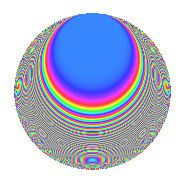# Properties

 Label 336.2.bjLevel 336 Weight 2 Character orbit bj Rep. character $$\chi_{336}(95,\cdot)$$ Character field $$\Q(\zeta_{6})$$ Dimension 32 Newforms 7 Sturm bound 128 Trace bound 7

# Related objects

## Defining parameters

 Level: $$N$$ = $$336 = 2^{4} \cdot 3 \cdot 7$$ Weight: $$k$$ = $$2$$ Character orbit: $$[\chi]$$ = 336.bj (of order $$6$$ and degree $$2$$) Character conductor: $$\operatorname{cond}(\chi)$$ = $$84$$ Character field: $$\Q(\zeta_{6})$$ Newforms: $$7$$ Sturm bound: $$128$$ Trace bound: $$7$$ Distinguishing $$T_p$$: $$5$$, $$13$$, $$19$$

## Dimensions

The following table gives the dimensions of various subspaces of $$M_{2}(336, [\chi])$$.

Total New Old
Modular forms 152 32 120
Cusp forms 104 32 72
Eisenstein series 48 0 48

## Trace form

 $$32q + O(q^{10})$$ $$32q + 8q^{13} - 12q^{21} + 28q^{25} + 4q^{37} - 12q^{45} + 8q^{49} - 48q^{57} - 8q^{61} - 96q^{69} + 20q^{73} - 36q^{81} - 48q^{85} - 24q^{93} - 40q^{97} + O(q^{100})$$

## Decomposition of $$S_{2}^{\mathrm{new}}(336, [\chi])$$ into irreducible Hecke orbits

Label Dim. $$A$$ Field CM Traces $q$-expansion
$$a_2$$ $$a_3$$ $$a_5$$ $$a_7$$
336.2.bj.a $$2$$ $$2.683$$ $$\Q(\sqrt{-3})$$ $$\Q(\sqrt{-3})$$ $$0$$ $$-3$$ $$0$$ $$-5$$ $$q+(-2+\zeta_{6})q^{3}+(-3+\zeta_{6})q^{7}+(3+\cdots)q^{9}+\cdots$$
336.2.bj.b $$2$$ $$2.683$$ $$\Q(\sqrt{-3})$$ $$\Q(\sqrt{-3})$$ $$0$$ $$-3$$ $$0$$ $$-1$$ $$q+(-2+\zeta_{6})q^{3}+(1-3\zeta_{6})q^{7}+(3-3\zeta_{6})q^{9}+\cdots$$
336.2.bj.c $$2$$ $$2.683$$ $$\Q(\sqrt{-3})$$ $$\Q(\sqrt{-3})$$ $$0$$ $$3$$ $$0$$ $$1$$ $$q+(2-\zeta_{6})q^{3}+(-1+3\zeta_{6})q^{7}+(3-3\zeta_{6})q^{9}+\cdots$$
336.2.bj.d $$2$$ $$2.683$$ $$\Q(\sqrt{-3})$$ $$\Q(\sqrt{-3})$$ $$0$$ $$3$$ $$0$$ $$5$$ $$q+(2-\zeta_{6})q^{3}+(3-\zeta_{6})q^{7}+(3-3\zeta_{6})q^{9}+\cdots$$
336.2.bj.e $$8$$ $$2.683$$ 8.0.8275904784.2 None $$0$$ $$-3$$ $$0$$ $$-6$$ $$q+(1-\beta _{1}-\beta _{5}+\beta _{6})q^{3}+(1-\beta _{2}-\beta _{3}+\cdots)q^{5}+\cdots$$
336.2.bj.f $$8$$ $$2.683$$ 8.0.303595776.1 None $$0$$ $$0$$ $$0$$ $$0$$ $$q+\beta _{1}q^{3}+(1-2\beta _{2}+\beta _{4}+2\beta _{6})q^{5}+\cdots$$
336.2.bj.g $$8$$ $$2.683$$ 8.0.8275904784.2 None $$0$$ $$3$$ $$0$$ $$6$$ $$q+\beta _{1}q^{3}+(-\beta _{1}-\beta _{4}-\beta _{7})q^{5}+(3+\cdots)q^{7}+\cdots$$

## Decomposition of $$S_{2}^{\mathrm{old}}(336, [\chi])$$ into lower level spaces

$$S_{2}^{\mathrm{old}}(336, [\chi]) \cong$$ $$S_{2}^{\mathrm{new}}(84, [\chi])$$$$^{\oplus 3}$$